# Android JNI 编程 - C语言基础知识 (一)

## 基础环境搭建

```# CLion remote docker environment (How to build docker container, run and stop it)

# ssh credentials (test user):

FROM centos:7

RUN sed -e 's|^mirrorlist=|#mirrorlist=|g' \ -e 's|^#baseurl=http://mirror.centos.org|baseurl=https://mirrors.tuna.tsinghua.edu.cn|g' \ -i.bak \ /etc/yum.repos.d/CentOS-*.repo

RUN yum -y update \ && yum -y install openssh-server \ make \ autoconf \ automake \ locales-all \ dos2unix \ ninja-build \ build-essential \ gcc \ gcc-c++ \ gdb \ clang \ cmake \ rsync \ tar \ python \ && yum clean all

RUN ssh-keygen -A

RUN ( \ echo 'LogLevel DEBUG2'; \ echo 'PermitRootLogin yes'; \ echo 'PasswordAuthentication yes'; \ echo 'Subsystem sftp /usr/libexec/openssh/sftp-server'; \ ) > /etc/ssh/sshd_config_test_clion

CMD ["/usr/sbin/sshd", "-D", "-e", "-f", "/etc/ssh/sshd_config_test_clion"] ```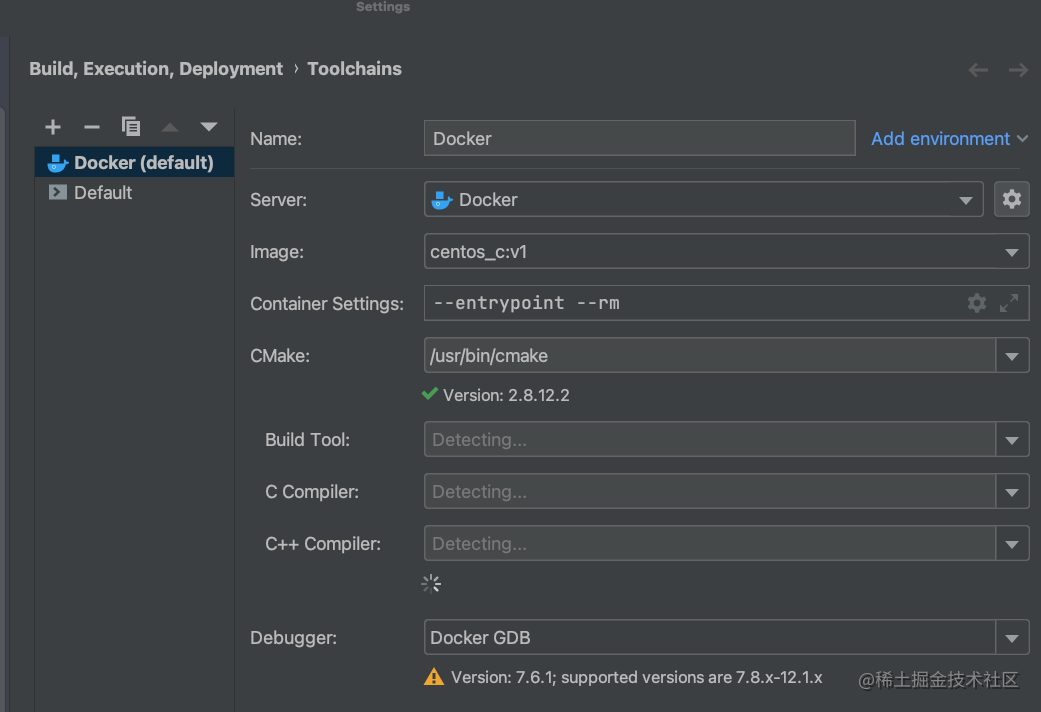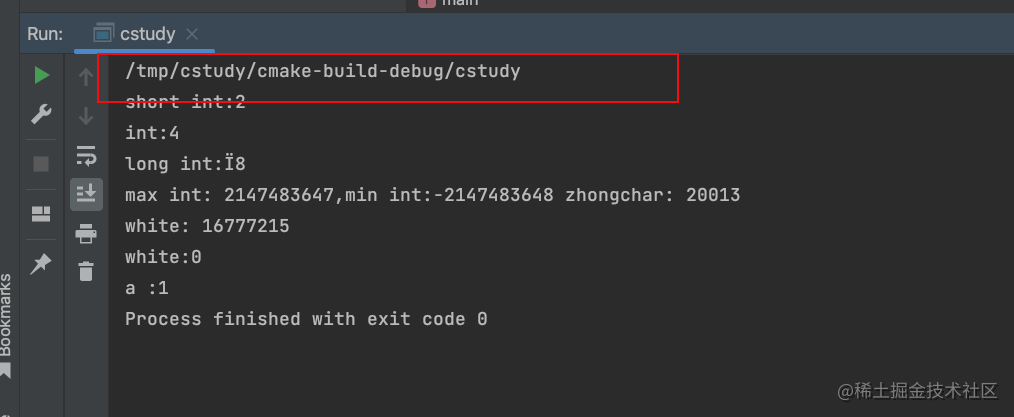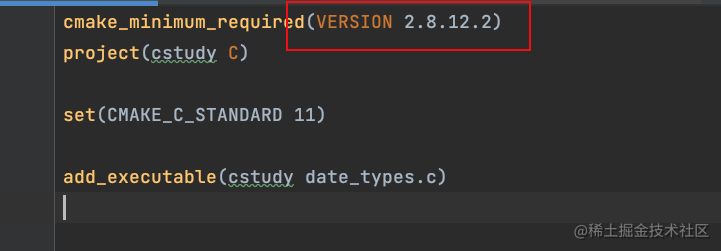``` cmake_minimum_required(VERSION 2.8.12.2) project(cstudy C)

set(CMAKE_C_STANDARD 11)

```

# include

void HandleVarargs(int arg_count,...) { // 用于获取变长参数 va_list args; // 开始遍历 va_start(args, arg_count); int j; for ( j = 0; j < arg_count; ++j) { // 取出对应参数 int arg = va_arg(args, int); printf("%d: %d \n", j, arg); } // 结束遍历 va_end(args); }

int main() { HandleVarargs(4,1,2,5,6); return 0; } ``` 在c中 写个变长参数的函数 真的累。。。 还要写这种模版代码

## 宏

### 头文件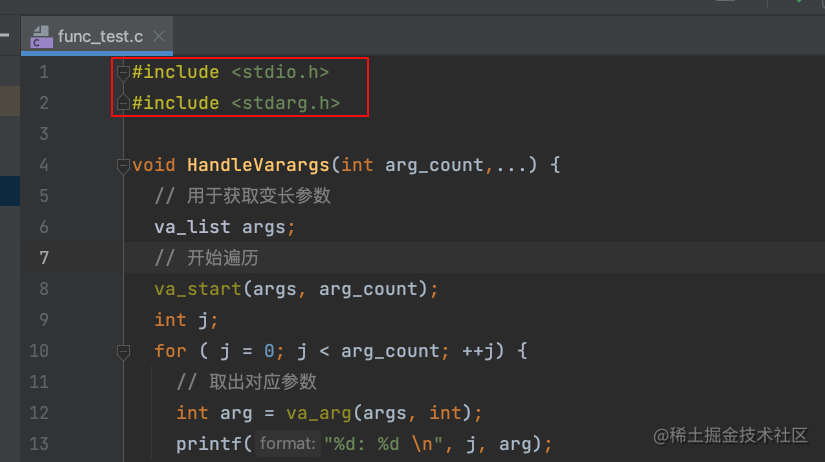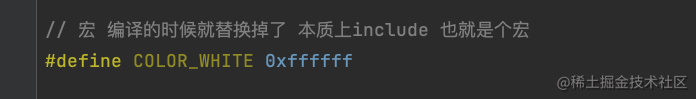### 自己写头文件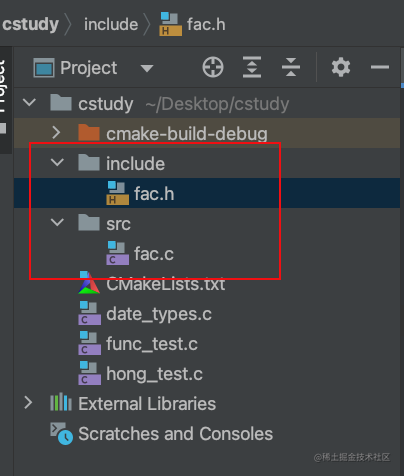``` // // Created by 吴越 on 2023/2/18. //

# define CSTUDY_INCLUDE_FAC_H_

int sum(int x,int y);

# endif //CSTUDY_INCLUDE_FAC_H_

```

``` // // Created by 吴越 on 2023/2/18. //

# include "../include/fac.h"

int sum(int x,int y){ return x+y; } ```

`add_executable(cstudy3 hong_test.c src/fac.c)`

```

# include "include/fac.h"

int main() { printf("sum: %d \n", sum(3, 5)); return 0; } ``` 就这么简单！

### <> 和 ““ 的区别

`include_directories("include")`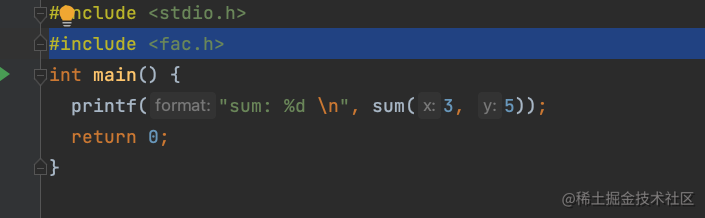```

# define MAX(a, b) a>b?a:b

int main() { printf("max : %d \n", MAX(1, 3)); printf("max : %d \n", MAX(1, MAX(4,3)));

return 0; } ``` 看下执行结果：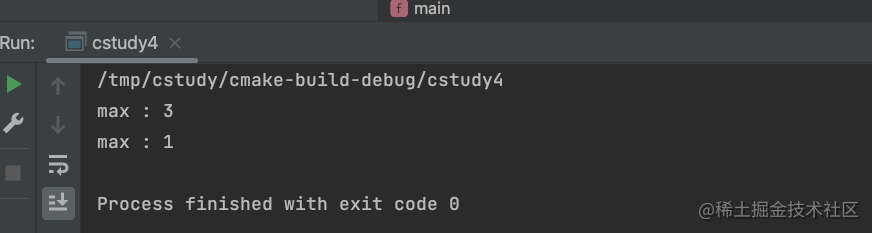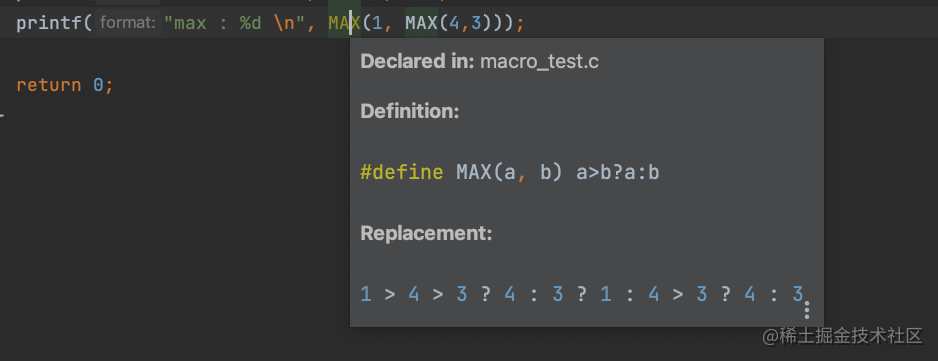```

# define MAX(a, b) (a) >(b) ? (a) :(b)

```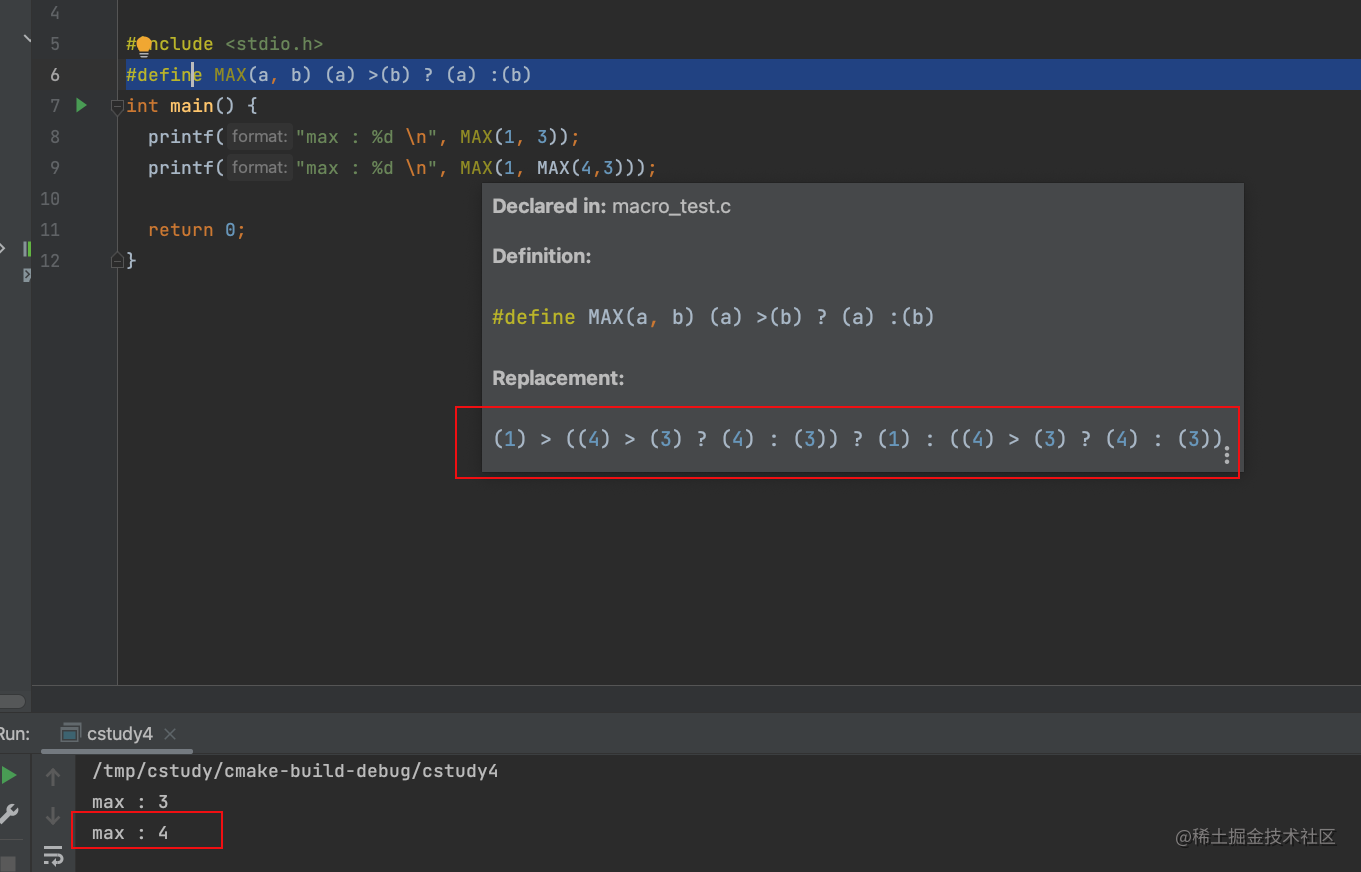```

# define MAX(a, b) (a) >(b) ? (a) :(b)

#define is_hex_char(c) \ ((c)>='0'&& (c)<='9') || \ ((c)>='a' && (c)<='f') int main() { printf("max : %d \n", MAX(1, 3)); printf("max : %d \n", MAX(1, MAX(4, 3))); printf("is hex : %d \n", is_hex_char('a')); return 0; } ```

### 条件编译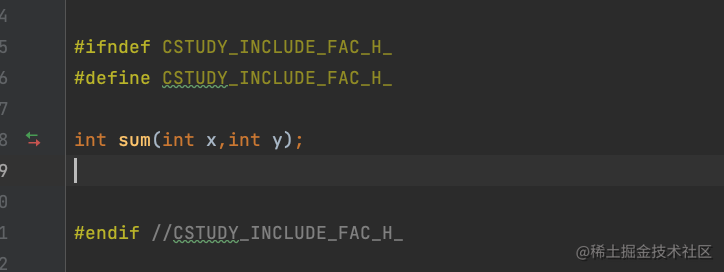```

void dump(){

# ifdef DEBUG

printf("debug info");

# endif

}

int main(){ dump(); return 0; } ``` 然后在cmake中写一下：

```add_executable(cstudy5 macro_test2.c) target_compile_definitions(cstudy5 PUBLIC DEBUG)```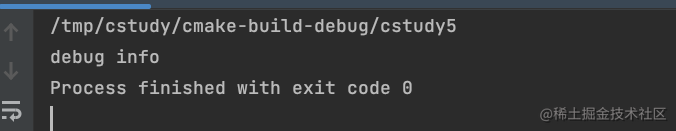```

# define PRINTFLINE(format, ...) printf(format"\n",##VA_ARGS)

void Printf(const char *format, ...) { va_list args; va_start(args, format); vprintf(format, args); printf("\n"); va_end(args); }

int main() { Printf("hello"); PRINTFLINE("world"); return 0; } ```

```

# define PRINT_INT(value) PRINTFLINE(#value": %d",value)

```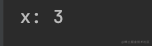```

# define PRINTFLINE(format, ...) printf("("FILE":%d) %s : "format"\n",LINE, FUNCTION, ##VA_ARGS)

```

## 字符串

### 字符串基本知识

c 语言中的字符串 必须以 null 也就是\0 为结尾

```

# include

int main(){ char string="wuyue"; char string2="wuyue"; PRINTFLINE("string: %s",string); PRINTFLINE("string2: %s",string2);

return 0; } ``` 来看下执行结果：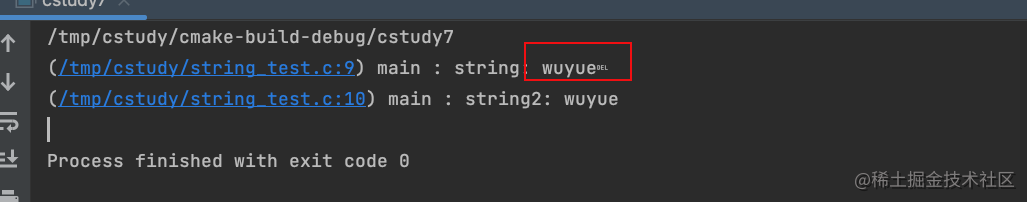## 指针

### 指针的只读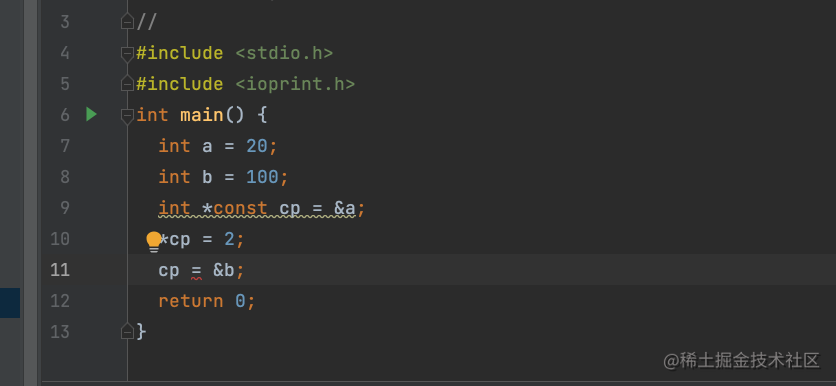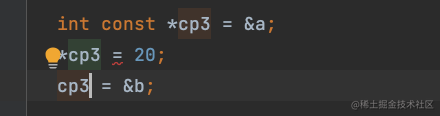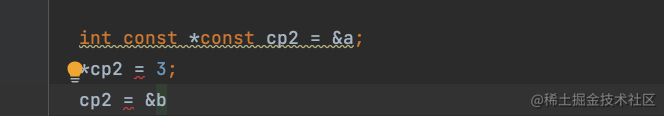const 如果修饰的是指针，则地址不能修改 const 如果修饰的是值，则值不能修改

```

# include

int main() {

int array = {0}; int pa= array; pa=2; (pa++)=3; (pa+2)=4; PRINT_INT_ARRAY(array,4); return 0; } ```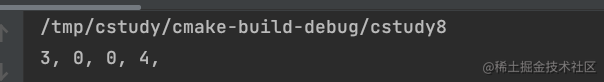### 指针参数作为返回值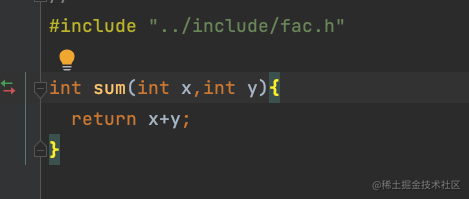```void sum2(int x, int y, int *result) { *result = x + y; }```

```

# include

int main() { int size = 10; int *array = malloc(sizeof(int) * size); int i; for (i = 0; i < size; ++i) { array[i]=i; } PRINT_INT_ARRAY(array,size) free(array); return 0;

} ```

``` void InitIntArray(int *a, int size, int defaultValue) { a = malloc(sizeof(int) * size); int i; for (i = 0; i < size; ++i) { a[i] = defaultValue; } }

int main() { int size = 10; int *a; InitIntArray(a,size,0); PRINT_INT_ARRAY(a, size) free(a); return 0; } ```

int main() { int size = 10; int *a; InitIntArray2(&a,size,0); PRINT_INT_ARRAY(a, size) free(a); return 0; } ```

```// 自动初始化 int *b = calloc(size, sizeof(int)); // 在之前的基础上，重新开辟一段空间，等于是扩大了 b = realloc(b, size * 2); if (b != NULL) { PRINTFLINE("分配成功"); PRINT_INT_ARRAY(b, size); } else{ PRINTFLINE("分配失败"); } return 0;```

### 函数指针

```void (*func)(int **aparams, int size, int defaultValue) = &InitIntArray2; size = 30; func(&a, size, 30); PRINT_INT_ARRAY(a, size);```

``` // f1是一个函数，这个函数的返回值 是一个int * 指针 int *f1(int,double );

// f2是一个函数指针，指向一个 参数为int，double 返回值是int的 函数 int (*f2) (int,double );

// f3和f2 一样，只不过返回值 是一个 int * 的函数 int (f3) (int,double );

// 函数的指针可以定义数组，但是函数不能定义数组 int (*f5[]) (int,double); ```

```// 定义这个函数指针的别名 typedef int (*Func)(int, int); int Add(int x, int y) { return x + y; }``` 然后去调用他

```Func func_1 = &Add; PRINTFLINE("result : %d" ,func_1(3, 4));```